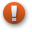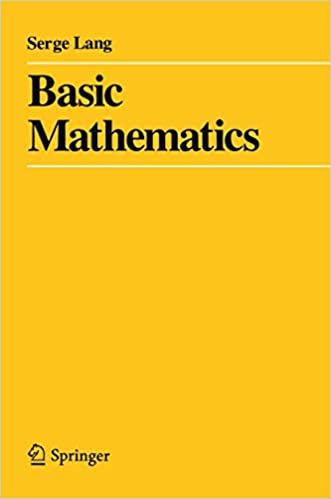# Basic Mathematics (Book)This article is a stub. You can help us by editing this page and expanding it.
Information Basic MathematicsSerge Lang English Springer 1 July 1988 496 0387967877 978-0387967875

The textbook Basic Mathematics by Serge Lang provides an overview of mathematical topics usually encountered through the end of high school/secondary school, specifically arithmetic, algebra, trigonometry, logic, and geometry. It serves as a solid review no matter how far along one may be in their studies, be it just beginning or returning to strengthen one's foundations.

Reading the Foreword and the Interlude is recommended for those unfamiliar with reading math texts.

Chapter/Section # Title Page #
PART I: ALGEBRA
Chapter 1: Numbers
1 The integers 5
3 Rules for multiplication 14
4 Even and odd integers; divisibility 22
5 Rational numbers 26
6 Multiplicative inverses 42
Chapter 2: Linear Equations
1 Equations in two unknowns 53
2 Equations in three unknowns 57
Chapter 3: Real Numbers
2 Real numbers: positivity 64
3 Powers and roots 70
4 Inequalities 75
Interlude: On Logic and Mathematical Expressions
2 Logic 94
3 Sets and elements 99
4 Notation 100
PART II: INTUITIVE GEOMETRY
Chapter 5: Distance and Angles
1 Distance 107
2 Angles 110
3 The Pythagoras theorem 120
Chapter 6: Isometries
1 Some standard mappings of the plane 133
2 Isometries 143
3 Composition of isometries 150
4 Inverse of isometries 155
5 Characterization of isometries 163
6 Congruences 166
Chapter 7: Area and Applications
1 Area of a disc of radius r 173
2 Circumference of a circle of radius r 180
PART III: COORDINATE GEOMETRY
Chapter 8: Coordinates and Geometry
1 Coordinate systems 191
2 Distance between points 197
3 Equation of a circle 203
4 Rational points on a circle 206
Chapter 9: Operations on Points
1 Dilations and reflections 213
2 Addition, subtraction, and the parallelogram law 218
Chapter 10: Segments, Rays, and Lines
1 Segments 229
2 Rays 231
3 Lines 236
4 Ordinary equation for a line 246
Chapter 11: Trigonometry
2 Sine and cosine 252
3 The graphs 264
4 The tangent 266
6 Rotations 277
Chapter 12: Some Analytic Geometry
1 The straight line again 281
2 The parabola 291
3 The ellipse 297
4 The hyperbola 300
5 Rotation of hyperbolas 305
PART IV: MISCELLANEOUS
Chapter 13: Functions
1 Definition of a function 313
2 Polynomial functions 318
3 Graphs of functions 330
4 Exponential function 333
5 Logarithms 338
Chapter 14: Mappings
1 Definition 345
2 Formalism of mappings 351
3 Permutations 359
Chapter 15: Complex Numbers
1 The complex plane 375
2 Polar form 380
Chapter 16: Induction and Summations
1 Induction 383
2 Summations 388
3 Geometric series 396
Chapter 17: Determinants
1 Matrices 401
2 Determinants of order 2 406
3 Properties of 2 x 2 determinants 409
4 Determinants of order 3 414
5 Properties of 3 x 3 determinants 418
6 Cramer's Rule 424
Index 429